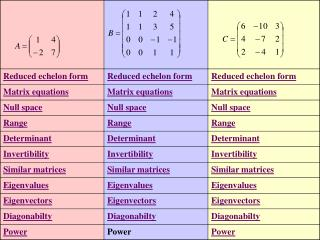DownloadDownload PresentationReduced echelon form

# Reduced echelon form

Télécharger la présentation## Reduced echelon form

- - - - - - - - - - - - - - - - - - - - - - - - - - - E N D - - - - - - - - - - - - - - - - - - - - - - - - - - -
##### Presentation Transcript

1. Reduced echelon form Because the reduced echelon form of A is the identity matrix,we know that the columns of A are a basis for R2 Return to outline

2. Matrix equations Because the reduced echelon form of A is the identity matrix: Return to outline

4. Every vector in the range of A is of the form: Is a linear combination of the columns of A. The columns of A span R2 = the range of A Return to outline

5. The determinant of A = (1)(7) – (4)(-2) = 15 Return to outline

6. Because the determinant of A is NOT ZERO, A is invertible (nonsingular) Return to outline

7. If A is the matrix for T relative to the standard basis,what is the matrix for T relative to the basis: Q is similar to A. Q is the matrix for T relative to the  basis, (columns of P) Return to outline

8. The eigenvalues for A are 3 and 5 Return to outline

11. A square root of A = A10 = Return to outline

15. The range of B is spanned by its columns. Because its null spacehas dimension 2 , we know that its range has dimension 2.(dim domain = dim range + dim null sp).Any two independent columns can serve as a basis for the range. Return to outline

16. Because the determinant is 0, B has no inverse. ie. B is singular Return to outline

17. If P is a 4x4 nonsingular matrix, then B is similar toany matrix of the form P-1 BP Return to outline

19. The null space of (2I –B)=The eigenspace belonging to 2 The null space of (0I –B)= the null space of B.The eigenspace belonging to 0= the null space of the matrix Return to outline

20. There are not enough independent eigenvectors to make a basis for R4 . The characteristic polynomial root 0 is repeated three times, but the eigenspace belonging to 0 is two dimensional. B is NOT similar to a diagonal matrix. Return to outline

24. The columns of the matrix span the range. The dimension of the null space is 1. Therefore the dimension of the range is 2. Choose 2 independent columns of C to form a basis for the range Return to outline

25. The determinant of C is 0. Therefore C has no inverse. Return to outline

26. For any nonsingular 3x3 matrix P, C is similar to P-1 CP Return to outline